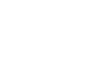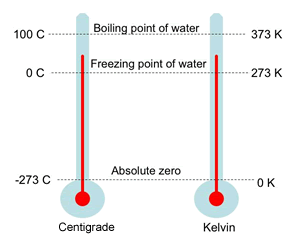What is the degree difference between Celsius And Kelvin temperature? - letsdiskussमयंक मानिक

Student-B.Tech in Mechanical Engineering,Mit Art Design and Technology University | Posted on | Science-Technology

What is the degree difference between Celsius And Kelvin temperature?

0
0

Manager at Amazon | Posted on

Celsius and Kelvin both are two units of measuring temperature. Celsius is commonly known as centigrade and mostly used unit system that is used to measure the temperature in daily use. On the other hand, Kelvin is mostly used in the scientific community.

The two measuring scales of temperature use different methods for the freezing and boiling points of water, and also use different sized degrees. Celsius is described in terms of the boiling (100°C) and freezing (0°C) points of water.

While Kelvin is mostly used in mathematical calculations that involves temperature. The degree difference between Kelvin and Celsius is "273.15". Converting from Kelvin to Celsius and vice versa is simple really, if you are converting from Celsius to Kelvin add 273.15 (K= °C + 273.15) and if you are converting to Kelvin from Celsius subtract 273.15 (°C= K-273.15).2
0